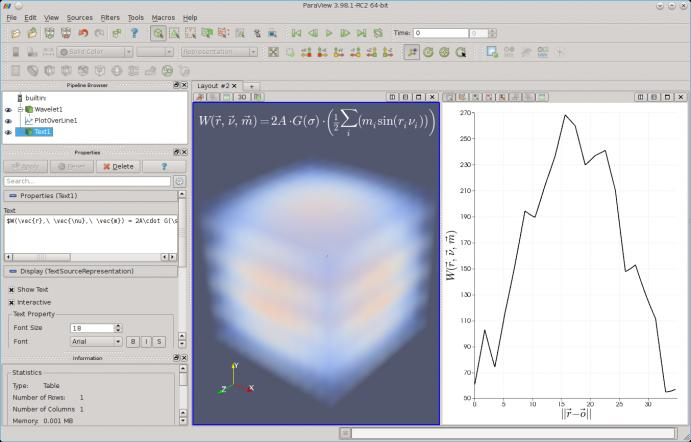# Writing Equations in ParaView 4.0 with MathText

June 10, 2013The ParaView UserVoice page is a place where users of the application can request features, and we pay attention to these requests and try to implement highly desired features when possible. One of the top requests has been for the ability to use LaTeX markup in ParaView text fields to draw mathematical equations as annotations and labels. I’m happy to announce that this request has been fulfilled, and ParaView 4.0 marks the first major release with this ability.The new release handles text entries containing a pair of dollar signs differently by rendering them as mathematical equations. The text between the dollar signs can be any valid MathText expression. While not quite LaTeX, MathText encompasses a large subset of the LaTeX markup language, and works much the same way. Users of the Python plotting toolkit matplotlib will already be familiar with MathText, as the convenient equation input functionality in matplotlib uses MathText. In fact, ParaView is actually using matplotlib under-the-hood for rendering these equations!

<

p dir=”ltr”>Entering mathematical symbols and equations in the new ParaView is easy as $\pi$ — to try this new feature out, download a copy of ParaView 4 today!

Tags:

## 2 comments to Writing Equations in ParaView 4.0 with MathText

1.Will Schroeder says:

It’s details like this that make a great application excellent! Nice job,

2.Raj Kumar Manna says:

Hi,

I want to use bold math like $bm{x/a}$ in Paraview text. Is it possible to inclue latex preamble like we do in matplotlib

plt.rc(‘text’, usetex=True)
plt.rcParams[‘text.latex.preamble’]=[r”usepackage{bm}”]

Is there any other way to include bold math?

Thanks
Raj